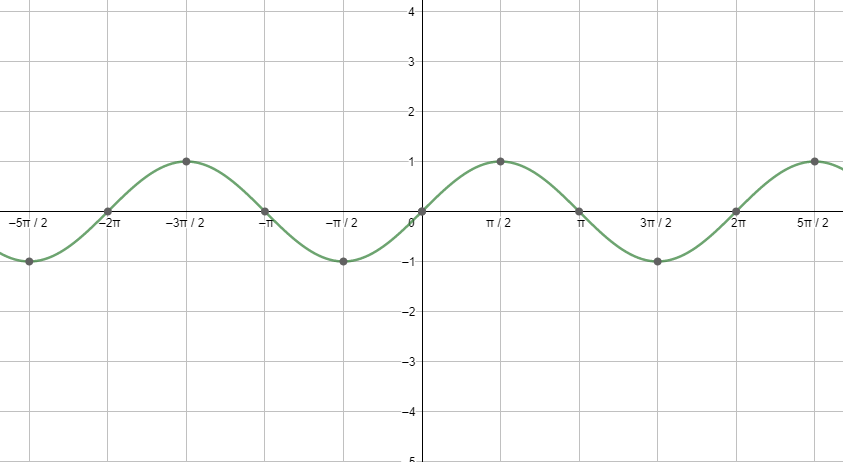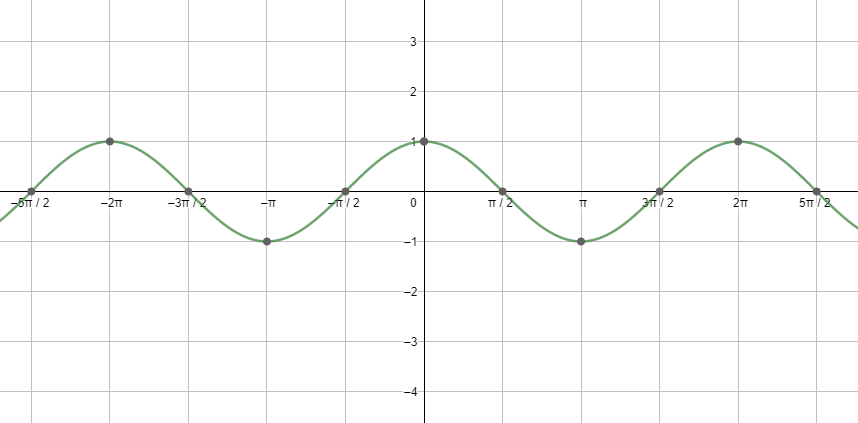QUESTION

# Evaluate the following Trigonometric function:$\dfrac{\sin 30{}^\circ -\sin 90{}^\circ +2\cos 0{}^\circ }{\tan 30{}^\circ \tan 60{}^\circ }$

Hint: All the angles used in the expression given in the question are standard angles so, we know the values of the trigonometric ratios given in the question; just use it and simplify to reach the answer.Looking at both the graphs, we can say that the graphs are repeating after a fixed period i.e. $2{{\pi }^{c}}$ . So, we can say that the fundamental period of the cosine function and the sine function is $2{{\pi }^{c}}=360{}^\circ$
$\dfrac{\sin 30{}^\circ -\sin 90{}^\circ +2\cos 0{}^\circ }{\tan 30{}^\circ \tan 60{}^\circ }$
Now we know $\sin 30{}^\circ =\dfrac{1}{2},\text{ sin90}{}^\circ \text{=1, cos0}{}^\circ \text{=1, tan30}{}^\circ \text{=}\dfrac{1}{\sqrt{3}},\text{ tan60}{}^\circ \text{=}\sqrt{3}$ . On putting these values in our expression, we get
$\dfrac{\dfrac{1}{2}-1+2}{\dfrac{1}{\sqrt{3}}\times \sqrt{3}}=\dfrac{1}{2}+1=\dfrac{3}{2}$
Therefore, the answer to the expression in the above question is $\dfrac{3}{2}$ .